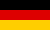# Bri's worldelectronics, programming and more

deutsch# PWM and function table calculation

This web app is suitable for PWM fading, sine and function table calculation for microcontrollers. The PWM and function tables are exported as C code. The button Show number table is used to show the number table values as a diagram, header and source file. The button Save header file and Save source file are used to save the number table code.

## PWM sine table settings

 table variable name data type data columns count number format Hex Decimal number of digits intend (number of spaces) table size (number of values)

 min. PWM value max. PWM value bits exponent n
 function y = f(x) = min. X value max. X value min. Y value max. Y value bits

## Diagram

``````
``````